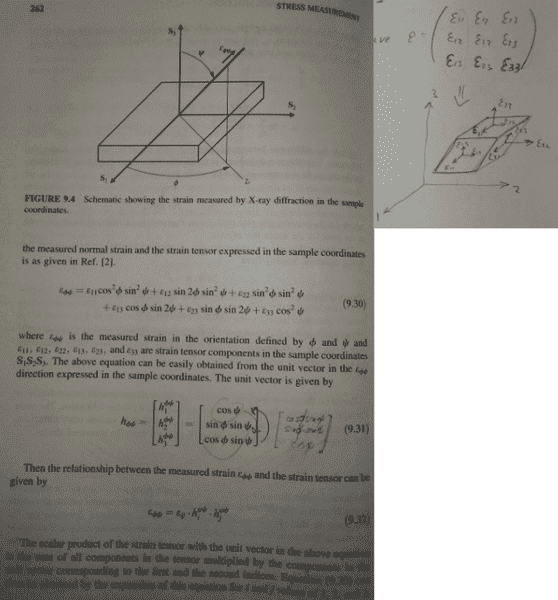# Question in linear algebra, derivation of a certain relation

• I
Hello good people,(notice the mistake in 9.31: cos(psi) switches places with cos(phi)sin(psi) to the best of my understanding)

Now, I am trying to derive 9.30 and for this, according to the book, we solve 9.32. The problem is I can not understand 9.32, the meaning of it.

I see it like this:

1) Group the strain tensor elements in each direction:
ST1=(s11+s21+s31)*e1 ; ST2=(s22+s12+s32)*e2 ; ST3=(s33+s13+s23)*e3
then the "s " stands for epsilon (strain) and the numbers are the strain element of the tensor.
e1,2,3 are unit vectors in S1,2,3 directions respectively.
2) Now, simply project the ST1,2,3 on the unit vector in the phi-psi direction, the h of 9.31. That way you get the magnitude of the strain in the phi-psi direction, which is what we want and what should be 9.30 according to the book. (of course s21=s12, s13=s31 & s23=s32)

I do it the like this:
component of ST1 on h = (h dot ST1) / |h| ; similarly for ST2 and ST3.
component of ST1 on h = (h dot ST1) / |h| = h1*(s11+s21+s31) ; similarly for ST2 and ST3.
As you can see I do not get the 9.30.

Where is my mistake?

Thank you very much

Anton